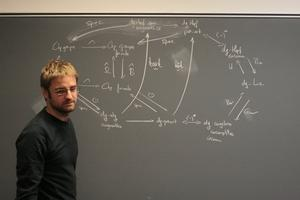Damien Calaque

Professor at IMAG (Univ Montpellier)

PI of ERC Project Derived Symplectic Geometry and Application

Email: damien.calaque[at]umontpellier.fr

Lecture Notes and Videos

Short lectures

• Vertex models and En-algebras: upside down renormalization (video)
• Deformation quantization with branes and multiple zeta values (beamer)
• Vertex models and En-algebras (video)
• En-algebras in Mathematical Physics (video 1, video 2)
• Two examples of fully extended TFTs (beamer of the first lecture)
• Derived symplectic geometry and classical Chern--Simons theory (video)
• Deformation quantization of shifted symplectic and Poisson structures (video)
• Hochschild cohomology of smooth algebraic varieties (.pdf)
• (in french) Algèbres de factorisation et modèles de réseaux (beamer)
• Lagrangian structures in derived symplectic geometry (.pdf)
• From associators to the Kashiwara-Vergne conjecture (video 1, video 2)
• Factorization algebras and formality theorems (video 1, video 2)
• Kontsevich formality theorem (video)
• Classical TFTs and symplectic structures on mapping spaces with boundary conditions (video)
• Einführungsvorlesung: Lie Theory and Algebraic Geometry (beamer, see also a video of the lecture)
• On the equivalence of two formality theorems, after Severa and Willwacher (video by Dror Bar-Natan / other talks of the conference are available on Bar-Natan's webpage)
• From Lie theory to algebraic geometry and back (.pdf, see also a video of a talk given on this subject at the conference Poisson 2010)
• (in french) Dualité de Van den Bergh et quantification par déformation, d'après Dolgushev (.pdf)
• Duflo isomorphism and Caldararu's conjecture (.pdf)
• (in french) Tranches et tubes (.pdf)
• Universal KZB equations, braids on the torus, and Cherednik algebras (slides in english and in french)
• Quantization of symplectic dynamical r-matrices and the quantum composition formula (.pdf)
• (in french) Cohomologie de Quillen pour les comodules sur un algébroïde de Hopf (.pdf)
• About the dynamical Yang-Baxter equation(s) (.pdf)

Lectures attended

• Superalgebra of differentiable functions and derived geometry - D. Roytenberg (.pdf)
• (in french) Déformations homogènes - P. Polesello (.pdf)
• (in french) Comparaison d'invariants quantiques - A. Virelizier (.pdf)
• (in french) Espaces de lacets et paramètres de Langlands - G. Ginot (.pdf)
Back to the main page